#NAME? error occurs when Excel cannot recognize something in the formula. It is a simple error that arises due to a spelling mistake in the formula name that you have typed or the reference provided in the formula doesn’t exist.

Follow this detailed tutorial on #NAME error in Excel and download this Excel workbook to practice along and  understand better:

### What is #NAME error in Excel?

When you are entering a formula, you might encounter an error, namely the Excel #NAME?. It is due to the fact that while entering the formula there might be a :

• Spelling error in Formula name
• Spelling error in Cell Range
• Spelling error in Named Range
• Text entered without quotes

It is a simple error to overcome and can be easily rectified. Let’s look into this in more detail.

### How to fix #NAME error in Excel?

In the entirety of the formula, any error in the spelling can lead to this error. Let’s learn how to rectify this in different situations.

Example 1- Formula name

When entering the name of the formula if the name is misspelled, then that can lead to the occurrence of this error.

In the above example, you can see that instead of writing =LEN(A7), we have typed =lan(A7). There is an error in the formula name, leading to the problem.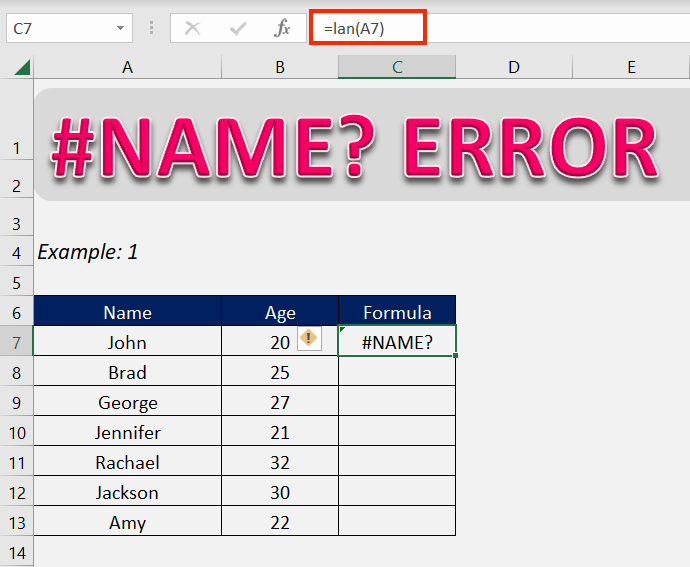Now you can see, simply correcting the name removes the error displayed.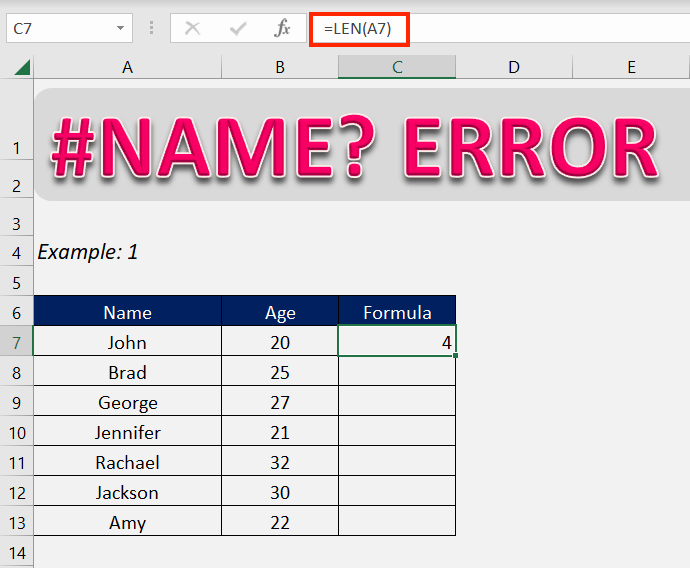The best way to not get #NAME excel error is to choose the formula in the drop-down list while typing the name of the formula. This makes sure that the formula name is not misspelled.

Example 2- Cell/Range name

When entering a formula that references a certain cell or range, misspelling the name of the cell or range can lead to this error.

Here we can see that the name of the cell is misspelled. Instead of =LEN(A7), it is typed as =LEN(AA).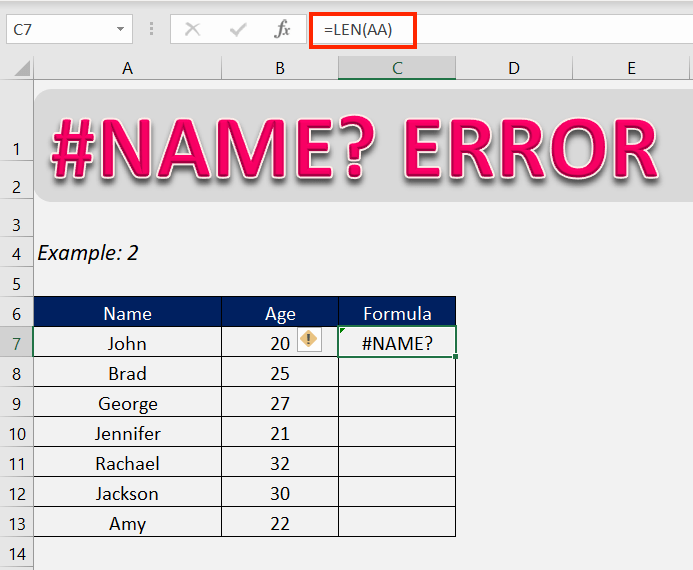To rectify this, simply type in the correct cell name.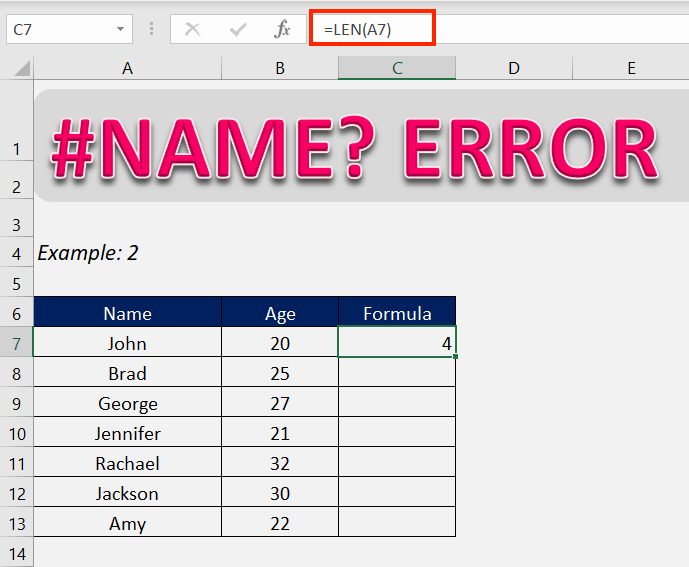A better way of avoiding the error is to select the cell when referencing in the formula. This will lead to always entering the correct cell name.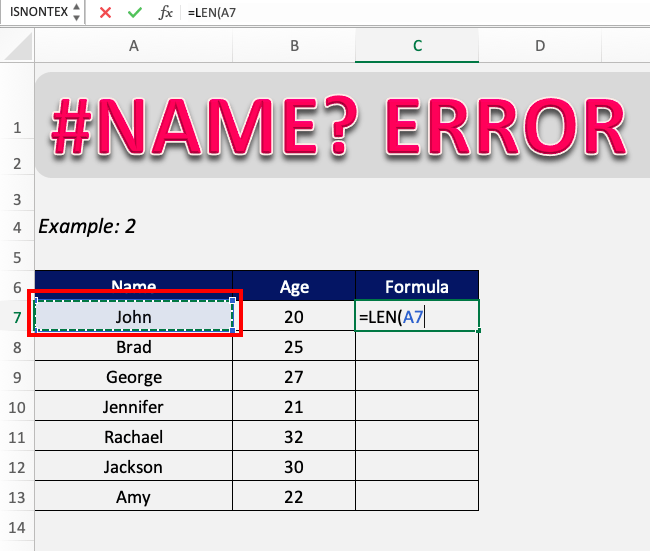NAME error in Excel can occur when entering a range. Check and correct the range name or select the entire range when entering the formula to rectify the error and prevent it.

Example 3- Named range

There can be particularly important ranges in your workbook that you have assigned a name to. When referencing this range in a formula, misspelling it can lead to the #NAME error in Excel.

Here we can see that the range referenced to in the formula is spelled agee when the correct name is Age.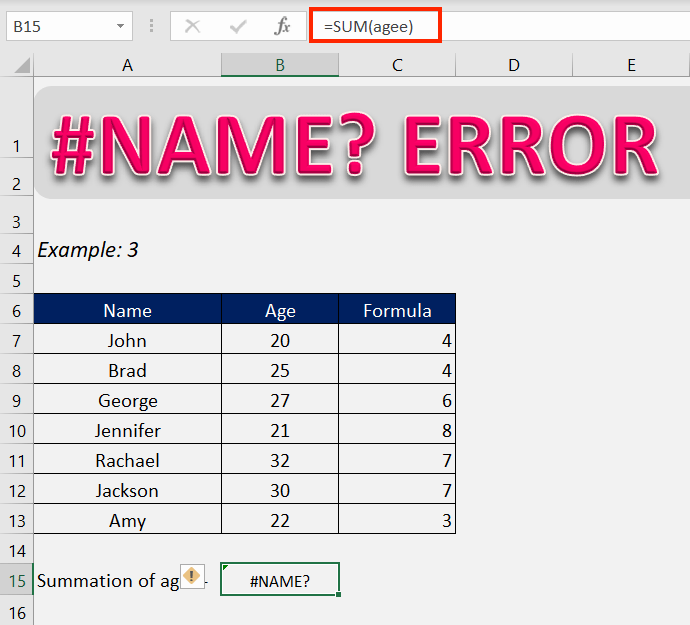Correcting the name of the range or simply selecting the range when referencing it in the formula will correct this error.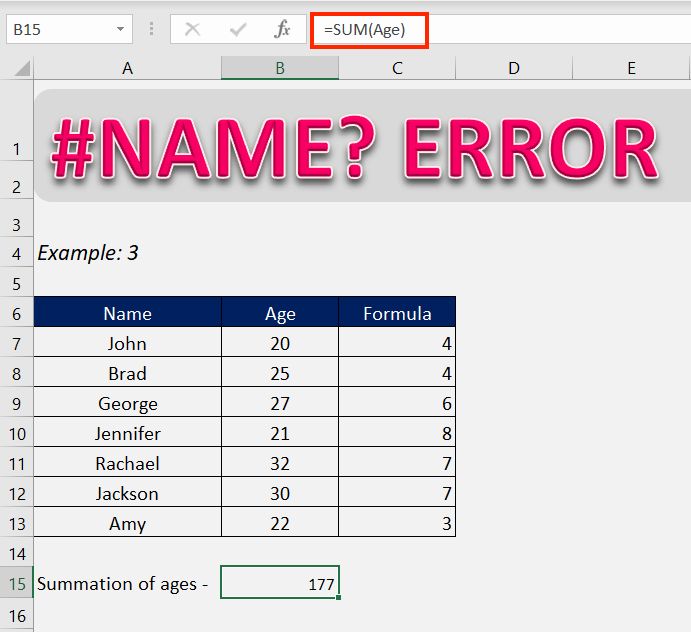Example 4- Text without quotes

Wherever a text is entered into a formula, it should be entered in quotes. When a text value is not confined between quotes, Excel reads it as either a formula or a named range/table.

If there is no formula or named range in your workbook that matches the text written in the formula, Excel will return the #NAME? Error.

Here we can see the text entered in the formula is not in quotes and hence Excel is returning it as an error.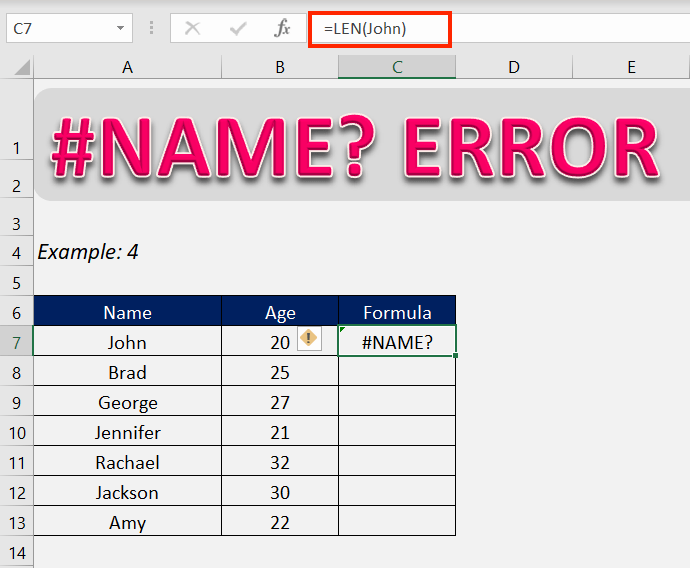Now that the text is written in quotes, Excel is returning the correct value of the formula.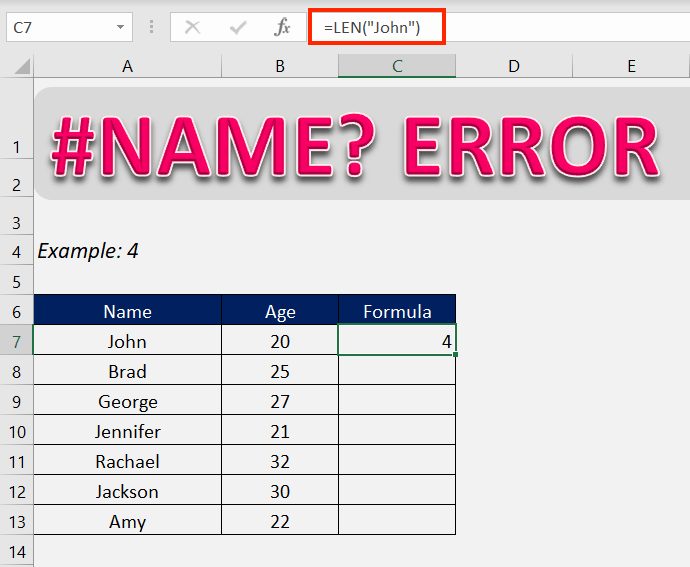### Conclusion

Now it should be clear that #NAME? Error, as evidenced by its name, is an error that occurs when there is a spelling error in different components of a formula, be it the name of the formula itself, or the values entered as the formula’s arguments.

After going through this exercise, we hope that your problems have been solved now.

Click here to learn about the Top 20 Common Errors that you may encounter while working on Excel.